# NCERT Solution for Class 9 Probability Exercise 15.1

In this page we have NCERT book Solutions for Class 9 Maths:Probability for EXERCISE 15.1 . Hope you like them and do not forget to like , social share and comment at the end of the page.

## Chapter 15 Ex 15.1

Question 1
In a cricket match, a bats woman hits a boundary 6 times out of 30 balls she plays. Find the probability that she did not hit a boundary.
Solution:
Let P is the event of hitting the boundary
n(P)=6
Let Q be the event of not hitting the boundary
n(Q)= 30- n(P)=24
Probability of not hitting the boundary= $\frac {n(Q)}{30}= \frac {24}{30}= \frac {4}{5}$

Question 2
1500 families with 2 children were selected randomly, and the following data were Recorded: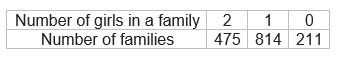Compute the probability of a family, chosen at random, having
(i) 2 girls
(ii) 1 girl
(iii) No girl
Also check whether the sum of these probabilities is 1.
Solution:
Total number of families n(S) =1500
Let P be the event of families having 2 girls
n(P)=475
Probability of event P= $\frac {n(P)}{n(S)} =\frac {475}{1500}=\frac {19}{60}$
Let Q be the event of families having 1 girls
n(Q)=814
Probability of event Q= $\frac {n(Q}{n(S)} = \frac {814}{1500}= \frac {407}{750}$
Let R be the event of families having no girls
n(R)=211
Probability of event R= $\frac {n(R)}{n(S)} = \frac {211}{1500}$
Let calculate the sum of all the above probabilities
$= \frac {19}{60} +\frac {407}{750} + \frac {211}{1500}$
=1

Question 3
In a particular section of class IX,40 students were asked about the month of their birth and following graph was prepared for the data so obtained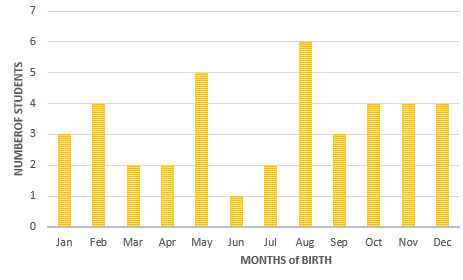Find the probability that a student of the class was born in August?
Solution:
Total number of students in the class n(S) =40
Let E be the event of getting born in August. Clearly from the histograph six students were born in the month of August out of 40 students of a particular section of class - IX.
N(E) =6
Probability that a student of the class was born in August
=N(E) /N(S)
$=\frac {6}{40}=\frac {3}{20}$

Question 4
Three coins are tossed simultaneously 200 times with the following frequencies of different outcomes.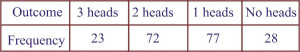If the three coins are simultaneously tossed again, compute the probability of 2 heads coming up.
Solution:
Since three coins are tossed 200 times, so the total number of trials is 200.
N(S)=200
Let E be the event of getting 2 heads coming up. It is clear from the table
N(E) =72
$= \frac {\text {N(E)}}{\text{N(S)}}$
$=\frac {72}{200}= \frac {9}{25}$

Question 5
An organization selected 2400 families at random and surveyed them to determine a relationship between income level and the number of vehicles in a family..The information gathered is listed in the table below :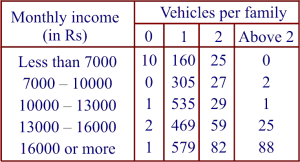Suppose a family is chosen. Find the probability that the family chosen is
(i) earning Rs 10000-13000 per month and owning exactly 2 vehicles.
(ii) earning Rs 16000 or more per month and owning exactly 1 vehicle.
(iii) earning less than Rs 7000 per month and does not own any vehicle.
(iv) earning Rs 13000 -16000 per month and owning more than 2 vehicles.
(v) owning not more than 1 vehicle
Solution:
The total number of families N(S) = 2400
(i)Let A be the event of Number of families earning Rs. 10000 - 13000 per month and owning exactly 2 vehicles
Then from the table
N(A)=29.
Therefore, P (families earning Rs 10000 - 13000 per month and owning exactly 2 vehicles)
$= \frac {\text {N(A)}}{\text{N(S)}}$
$=\frac {29}{2400}$

(ii)Let B be the event ofNumber of families earning Rs 16000 or more per month and owning exactly 1 vehicle
N(B) = 579.
Therefore P (Families earning Rs 16000 or more per month and owning exactly 1 vehicle)
$= \frac {\text {N(B)}}{\text{N(S)}}$
$=\frac {579}{2400}$

(iii)Let C be the event of Number of families earning less than Rs. 7000 per month and does not own any vehicle
N(C) = 10
Therefore, P (Families earning less than Rs 7000 per month and does not own any vehicle)
$= \frac {\text {N(C)}}{\text{N(S)}}$
$= \frac {10}{2400}$
$=\frac {1}{240}$

(iv)Let D be the event of Number of families earning Rs 13000 - 16000 per month and owning more than 2 vehicles
N(D)= 25
Therefore, P (Families earning Rs 13000 - 16000 per month and owning more than two vehicles)
$= \frac {\text {N(D)}}{\text{N(S)}}$
$=\frac {25}{2400}=\frac {1}{96}$

(v)Let R be the event of Number of families owning not more than 1 vehicle N(R) = Families having no vehicle + Families having 1 vehicle = (10 + 0 + 1 + 2 + 1) + (160 + 305 + 535 + 469 + 579) = 14 + 2048 = 2062
P (Families owning not more than 1 vehicle) $= \frac {\text {N(R)}}{\text{N(S)}}$
$=\frac {2062}{2400}=\frac {1031}{1200}$

Question 6
A teacher wanted to analyse the performance of two sections of students in a mathematics test of 100 marks. Looking at their performances, she found that a few students got under 20 marks and a few got 70 marks or above. So she decided to group them into intervals of varying sizes as follows: 0 - 20, 20 - 30, . . ., 60 - 70, 70 - 100. Then she formed the following table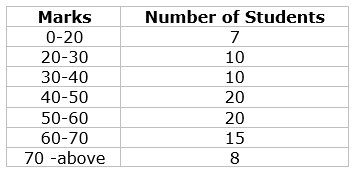(i) Find the probability that a student obtained less than 20% in the mathematics test.
(ii) Find the probability that a student obtained marks 60 or above.
Solution:
Total number of students in mathematics N(S)= 90.
(i)Let A be the event of student obtained less than 20% in the mathematics test.
Clearly, from the given table, the number of student who obtained less than 20% marks in the mathematics test N(A)= 7.
P (a student obtaining less than 20% marks) = $\frac {\text {N(A)}}{\text{N(S)}}= \frac {7}{90}$

(ii)Let B be the event of student obtained marks 60 or above.Clearly, from the given table, number of students who obtained marks 60 or above.
N(B)= (students in 60 - 70) + (students above 70)
= 15 + 8 = 23
Therefore, P (a student obtaining marks 60 and above) =$\frac {\text {N(B)}}{\text{N(S)}}=\frac {23}{90}$

Question 7
To know the opinion of the students about the subject statistics, a survey of 200 students was conducted. The data is recorded in the following table.Find the probability that a student chosen at random
(i) likes statistics
(ii) does not like it.
Solution
The total number of students
N(S)= 200
(i)Let A be the event of student chosen at random likes statistics
N(A)=135
P (a student likes statistics)
$=\frac {\text {N(A)}}{\text{N(S)}}$ $=\frac {135}{200}= \frac {27}{40}$

(ii) Let B be the event of student chosen at random does not likes statistics
N(B)=65
P (a student does not like statistics)
$=\frac {\text {N(B)}}{\text{N(S)}}$ $=\frac {65}{200}$
$=\frac {13}{40}$

Question 8
The distance (in km) of 40 engineers from their residence to their place of work were found as follows:
 5 3 10 20 25 11 13 7 12 31 19 10 12 17 18 11 32 17 16 2 7 9 7 8 3 5 12 15 18 3 12 14 2 9 6 15 15 7 6 12
(i) less than 7 km from her place of work?
(ii) more than or equal to 7 km from her place of work?
(iii)within ½ km from her place of work?
Solution:
Total number of engineers N(S) = 40
(i)Let A be the event of engineer living less than 7 km from her place of work
N(A) = 9
Therefore P (an engineer lives less than 7 km from her place of work)
$=\frac {\text {N(A)}}{\text{N(S)}}$
$=\frac {9}{40}$

(ii)Let B be the event of engineer living more than or equal to 7 km from her place of work
N(B) = 31
Therefore P (an engineer lives less than or equal to 7 km from her place of work)
$=\frac {\text {N(B)}}{\text{N(S)}}$
$=\frac {31}{40}$

(iii)Let C be the event of engineer living within ½ km from her place of work
N(C) = 0
Therefore P (an engineer lives with 12 km from her place of work) =0

Question 9
Activity : Note the frequency of two-wheeler, three -wheeler and four -wheeler going past during a time interval,in front of your school gate. Find the probability that any one vehicle out of the total vehicles you have observed is a two wheeler.
Solution:
Everybody will have separate solution as data will be different. Let me demonstrate the solution with one set of data
After observing the frequency of vehicles at certain time, Here is the data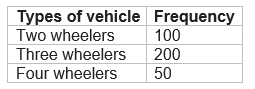So total number of vehicle
N(S)= 100+200+50=350
Number of two wheelers
N(T)=100
$\text {Probability}= \frac {\text {N(T)}}{\text{N(S)}}= \frac {100}{350}=\frac {2}{7}$

Question 10
Activity : Ask all the students in your class to write a 3-digit number. Choose any student from the room at random. What is the probability that the number written by her/him is divisible by 3? Remember that a number is divisible by 3, if the sum of its digits is divisible by 3.
Solution
Activity problem : Do it yourself

Question 11
Eleven bags of wheat flour, each marked 5 kg , actually contained the following weights of flour (in kg) : 4.97 5.05 5.08 5.03 5.00 5.06 5.08 4.98 5.04 5.07 5.00
Find the probability that any of these bags chosen at random contains more than 5kg of flour.
Solution
Total number of wheat bags
N(S)= 11
let A be the event of Number of bags having more than 5 kg
N(A) = 7
Therefore P(a bag contains more than 5kg ) = $\frac {\text {N(A)}}{\text{N(S)}} =\frac {7}{11}$

Question 12
A study was conducted to find out the concentration of sulphur dioxide in the air in parts per million (ppm) of a certain city. The data obtained for 30 days is as follows
 0.03 0.08 0.08 0.09 0.04 0.17 0.16 0.05 0.02 0.06 0.18 0.2 0.11 0.08 0.12 0.13 0.22 0.07 0.08 0.01 0.1 0.06 0.09 0.18 0.11 0.07 0.05 0.07 0.01 0.04
you were asked to prepare a frequency distribution table, regarding the concentration of sulphur dioxide in the air in parts per million of a certain city for 30 days. Using this table, find the probability of the concentration of sulphur dioxide in the interval 0.12 - 0.16 on any of these days.
Solution:
Frequency distribution
 Interval Frequency .01-.04 5 .04-.08 11 .08 - .12 7 .12- .16 2 .16-.20 4 .20-.24 1
Total number of days n(S) = 30 let A be the event of Concentration of sulphur dioxide in 0.12 - 0.16 on any day

N(A)= 2
Therefore,required probability= $\frac {2}{30}=\frac {1}{5}$

Question 13
The blood groups of 30 students of Class VIII are recorded as follows:
A, B, O, O, AB, O, A, O, B, A, O, B, A, O, O,
A, AB, O, A, A, O, O, AB, B, A, O, B, A, B, O.
You were asked to prepare a frequency distribution table regarding the blood group of 30 students of a class. Use this table to determine the probability that a student of this class,selected at random,has blood group AB.
Solution
Frequency distribution
 Blood Group Frequency A 9 B 6 O 3
Total number of students
N(S)= 30
let E be the event of Number of students having blood group AB
N(E) = 3
Therefore required probability=$\frac {\text {N(E)}}{\text{N(S)}} = \frac {3}{30} = \frac {1}{10}$

## Summary

1. NCERT solutions for class 9 maths chapter 15 Probability Ex 15.1 has been prepared by Expert with utmost care. If you find any mistake.Please do provide feedback on mail. You can download the solutions as PDF in the below Link also
2. This chapter 15 has total 1 Exercise 15.1. This is the only exercise in the chapter.

• Notes
• NCERT Solutions & Assignments